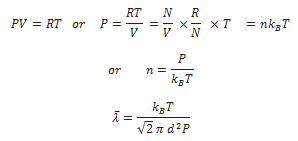The mean free path l is the average distance covered by a molecule between two successive collisions: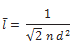Where n is the number density and d the diameter of the molecule.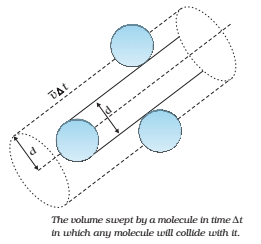Let n be the number of molecules per unit volume.

Number of collisions suffered by the molecule A in time ∆t = volume of the cylinder swept by molecule A in time ∆t × number of molecules per unit volume =

Mean free path of a gas molecule,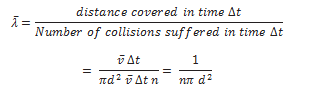In the above derivation, we have assumed the other molecules to be at rest. Taking into consideration the motion of all the gas molecules, the mean free path comes out to be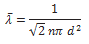If m is the mass of each gas molecule, then the density of the gas is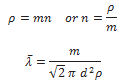For one mole of a gas,